# Circular Sector Calculator

## Circular sector definition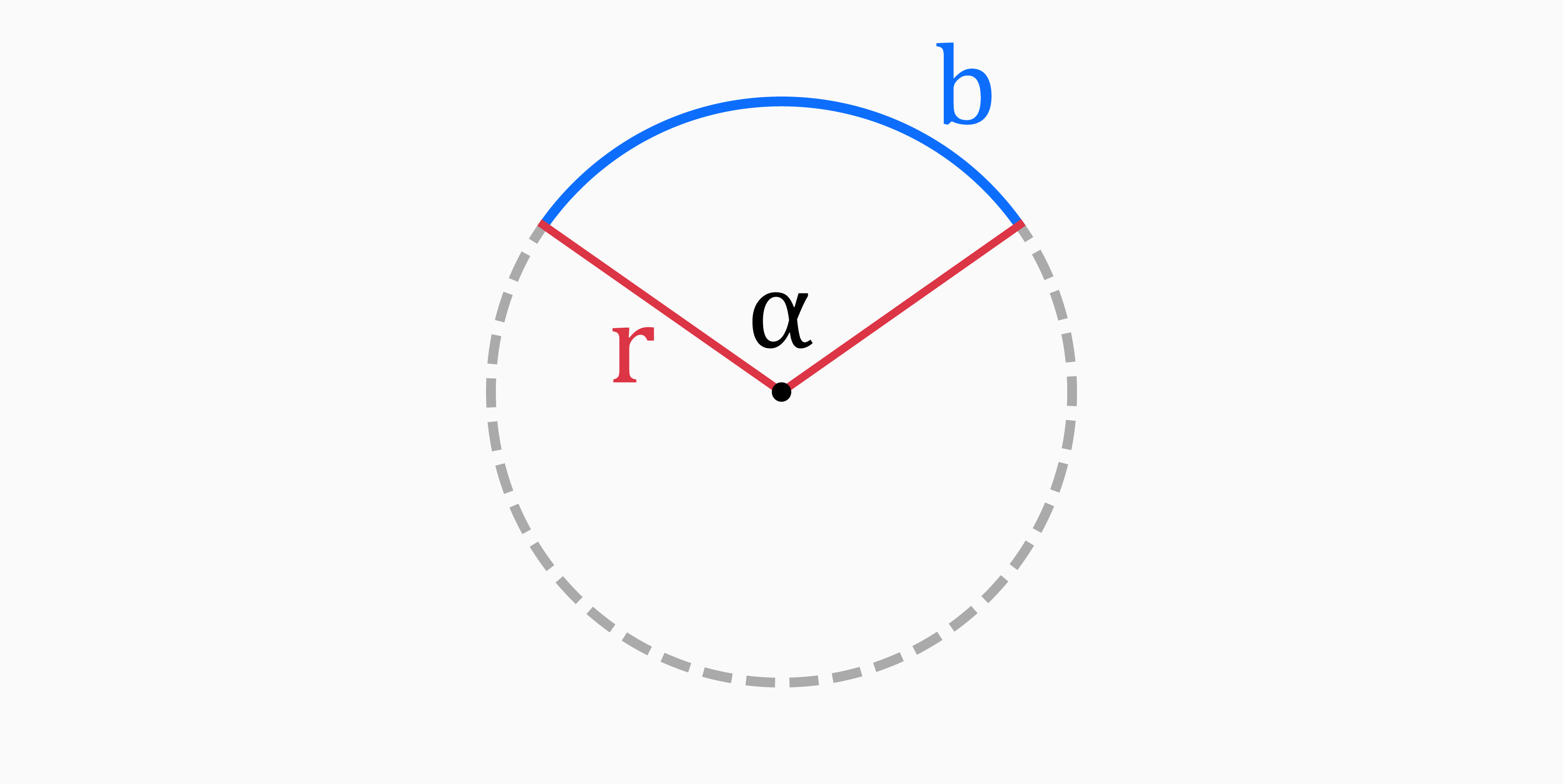A circle sector is a portion of a circle enclosed between two radii and an arc.

In other words, it is a part of a circle that is bounded by two radii and an arc, where the arc is a fraction of the circumference of the circle.

The angle formed by the two radii that bound the sector is called the central angle of the sector.

## Parts of a circular sector

The five main parts of a circle are radius (r), arc length (b), central angle (α), area (A) and perimeter (P).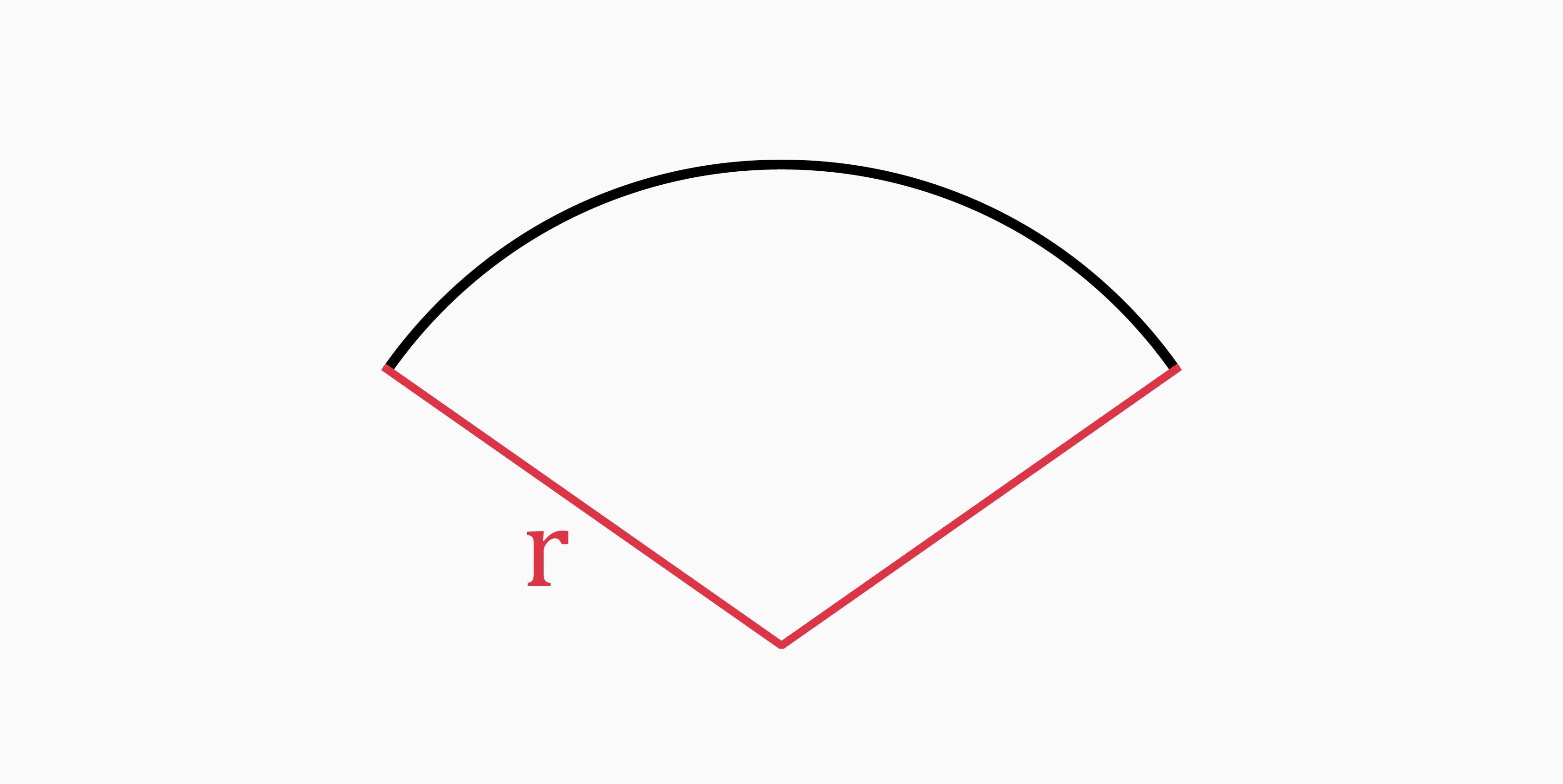The radius of a circular sector is a straight line from the center of the circle to the circumference. It is usually denoted by "r". It can be found with the following formulas:

• If you know the central angle and arc length:

`r = b / α` (when α is in radians)

`r = b / α * (180 / π)` (when α is in degrees)

• If you know the arc length and perimeter:

`r = (P - b) / 2`

• If you know the arc length and area:

`r = 2 * A / b`

• If you know the area and central angle:

`r = √(2 * A / α)` (when α is in radians)

`r = √(2 * A / α * (180 / π))` (when α is in degrees)

### Arc length (b)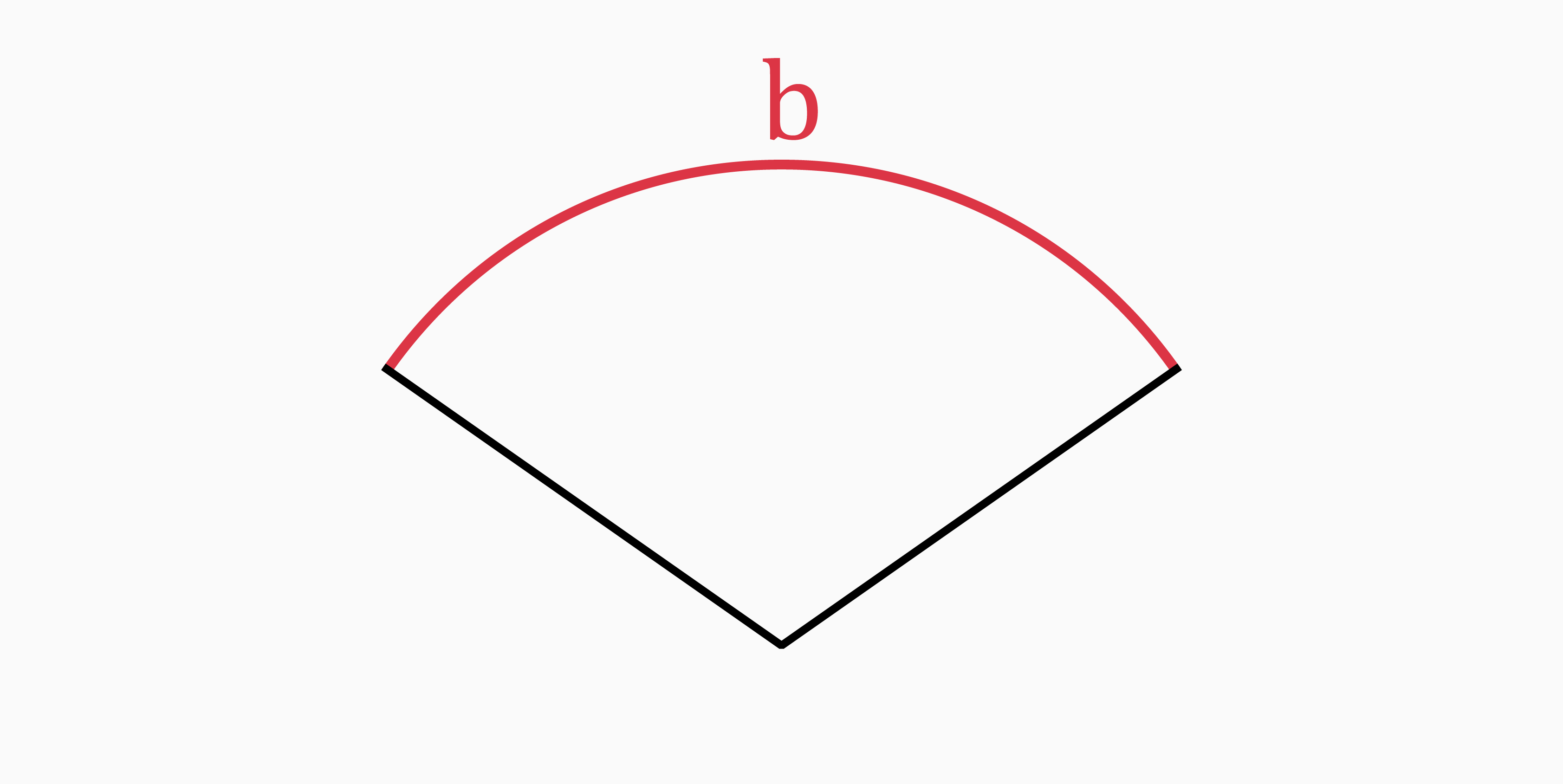The arc length of a circular sector is the portion of the circumference that makes up the sector. In our tool it is denoted by "b".

You can determine it with these formulas:

• If you know the radius and central angle:

`b = r * α` (when α is in radians)

`b = r * α * (π / 180)` (when α is in degrees)

• If you know the radius and area:

`b = 2 * A / r`

• If you know the radius and perimeter:

`b = P - 2 * r`

### Angle (α)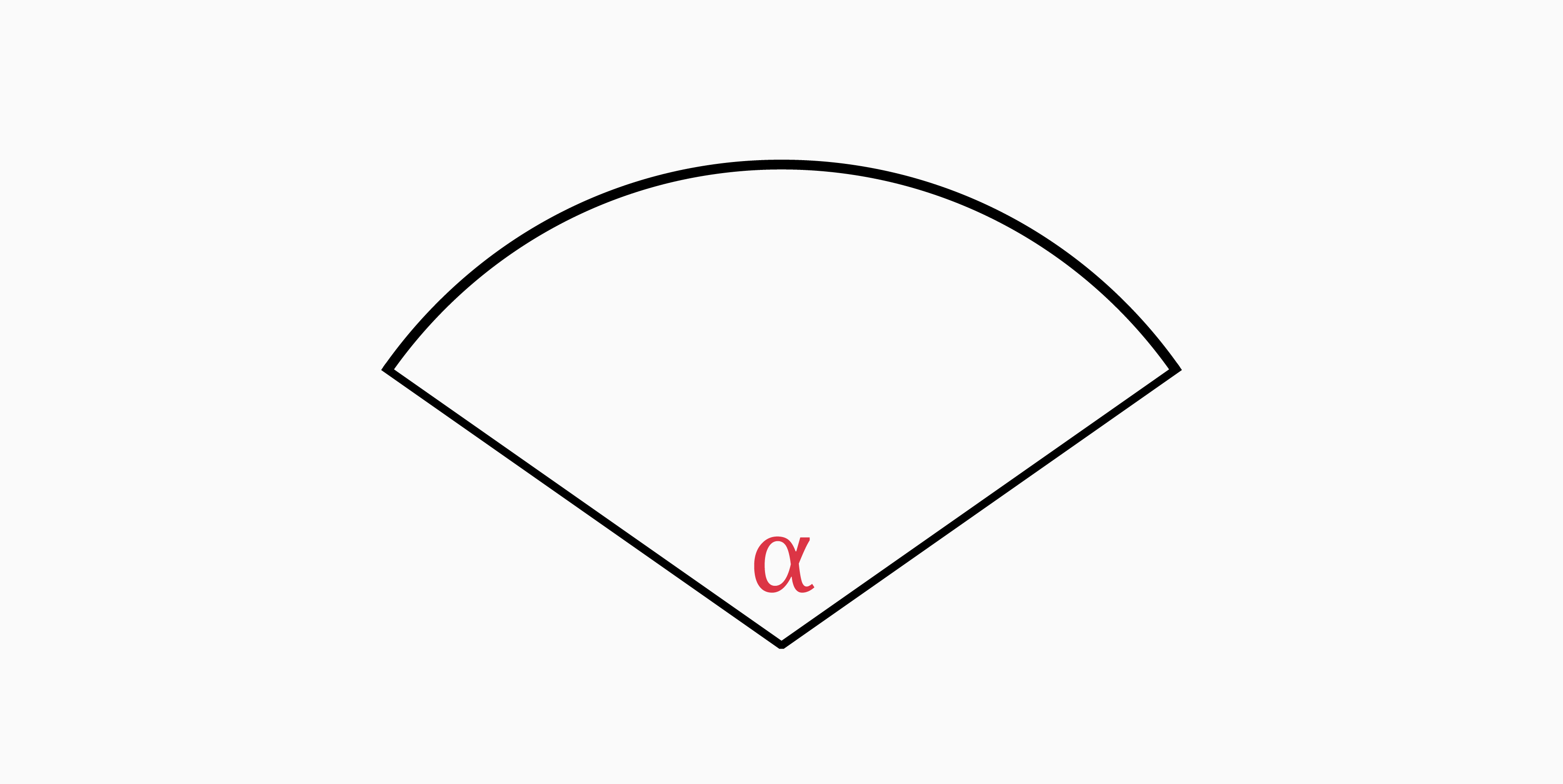The angle of a circular sector, denoted by "α", is the central angle formed by two radii of a circle that define the boundaries of the sector. The size of the angle determines the size of the sector: the larger the angle, the larger the sector.

If you know the radius and arc length, you can calculate it as follows:

`α = b / r` (in radians)

`α = b / r * (180 / π)` (in degrees)

### Area (A)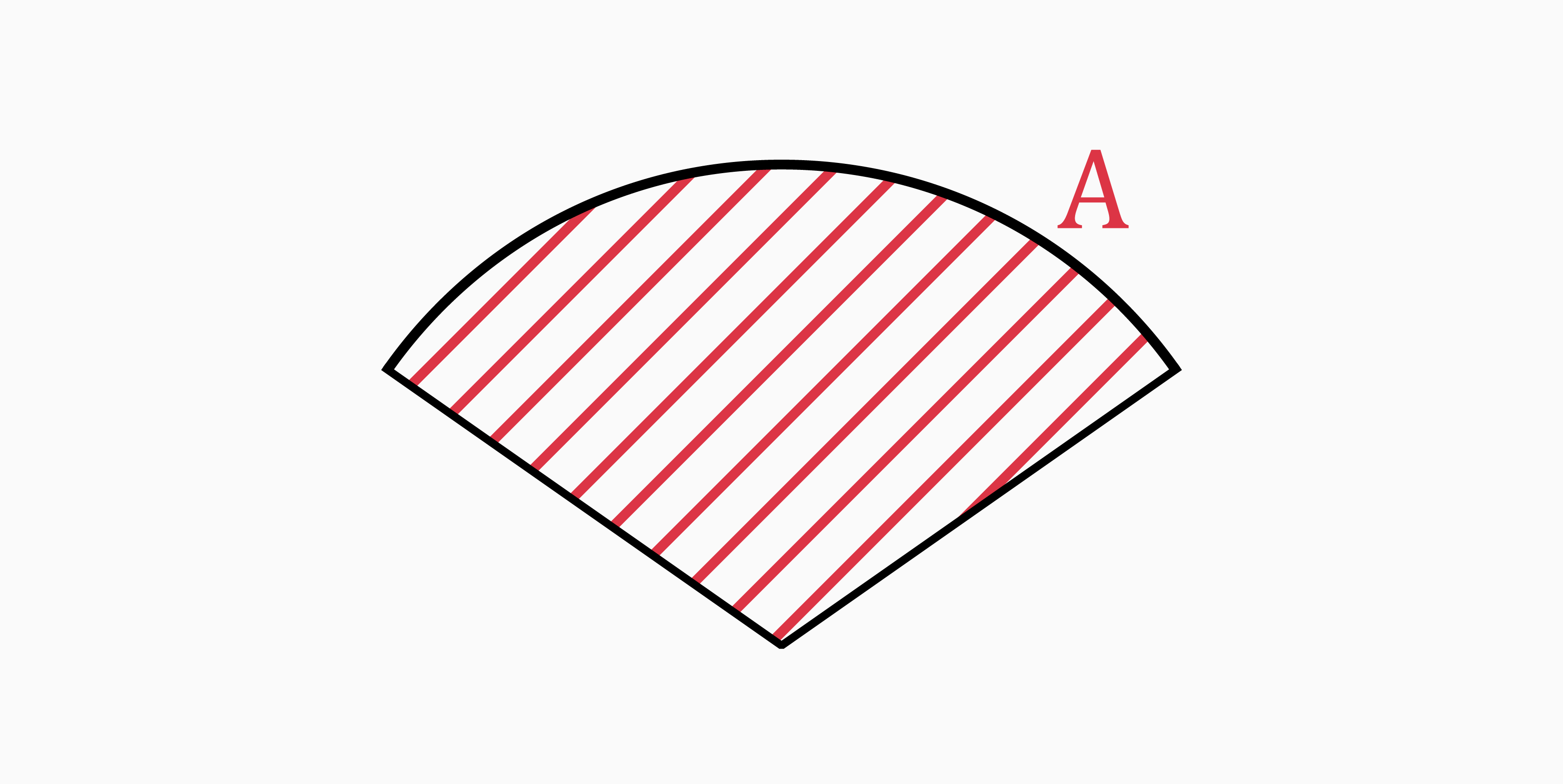The area of a circular sector is the region enclosed by the sector. To find the area of a circular sector, you can use the following formulas:

• If you know the radius and arc length:

`A = b * r / 2`

• If you know the radius and central angle:

`A = α * r² / 2` (when α is in radians)

`A = α * r² / 2 * (π / 180)` (when α is in degrees)

### Perimeter (P)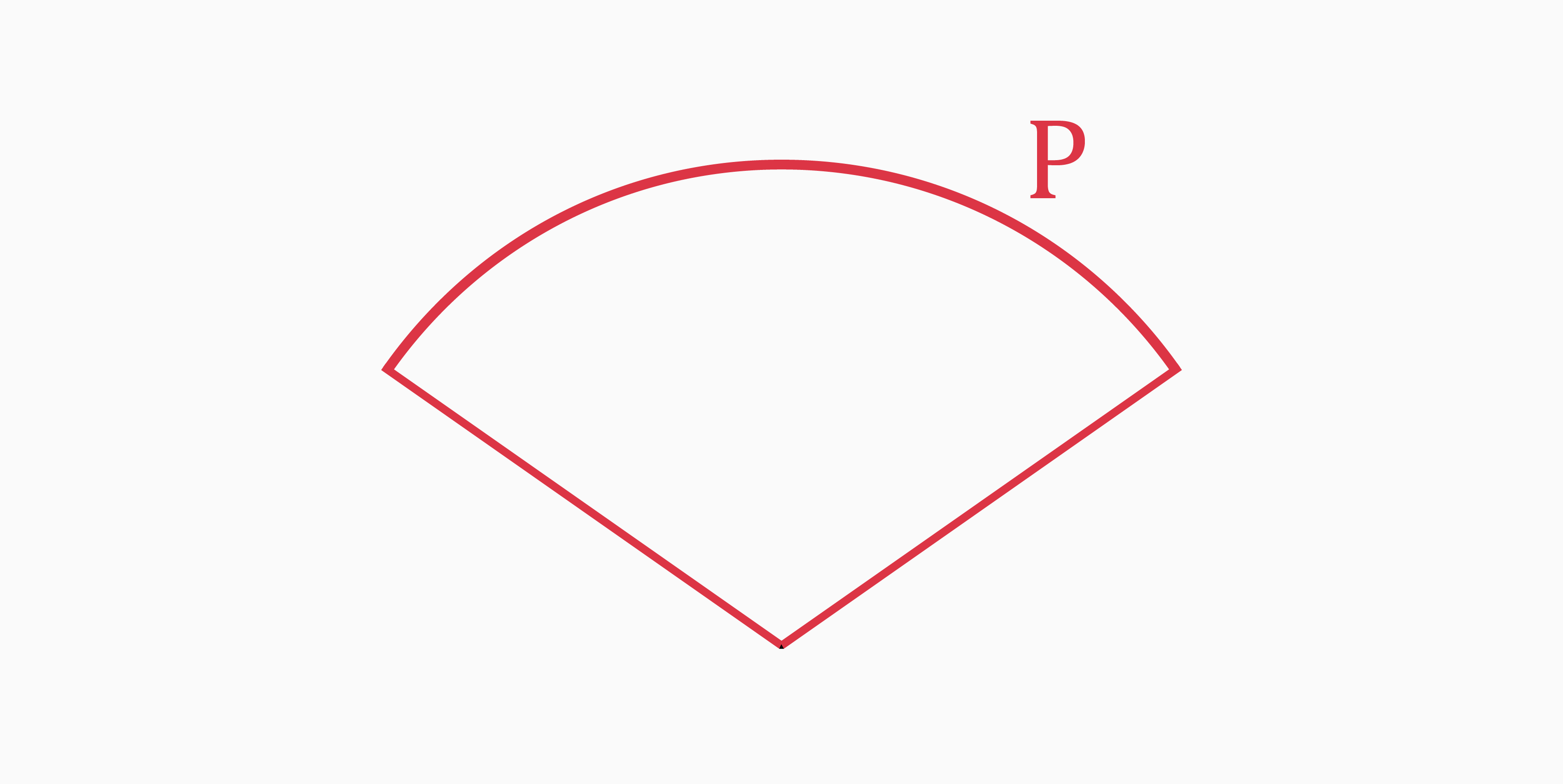The perimeter of a circular sector is the distance around the edge of the sector which is formed by an arc and two radii. It is usually denoted by "P".

The formula to calculate the perimeter of a circular sector is given by:

`P = 2 * r + b`